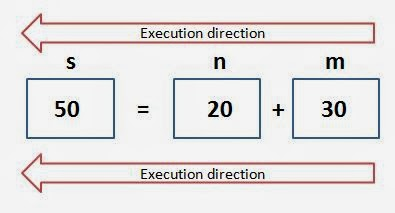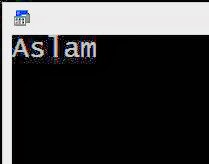# Declaring and Using a Variable in C

In previous lesson, we learnt how to display or show a text string.
Now let us see how to declare a value and how to print or display the declared value as output.

Let us learn these with an example program:
[In this lesson there are many example programs, you should work with your compiler to understand actual working of codes]

 #include          #include      void main()      {        int n;        n = 10;        printf(“%d”,n);        getch();       }

Explanation:

The lines #include<stdio.h>, #include<conio.h> and getch() were discussed already on previous lesson.

int n; – int stands for integer. Here computer will prepare a small memory with variable ‘n’ to store only integers (decimal numbers).n = 10; – Here computer will put a value of 10 to the above prepared memory n.printf(“%d”,n); %d (says module d) is denote a place to display(where to show) a decimal number and n replace the place of %d to display. That is in C, just printf(n) will not produce an output with 10. If you see the code %d inside a double quotes, then you have to think there should be a decimal number to display instead of it. That is the variable (here that variable is n) or  its value (here the value of n is 10) after comma  replace the place of %d.It prints the value of n, so output will be 10

Another program to declaring two variables, then assigning values to variables and printing each value:

 #include #include void main() { int n; int m; n = 10; m = 20; printf(“%d and %d are numbers”,n,m); getch(); }

Explanation:

int n;int m;

-Declaring n and m as integers.

n = 10;m = 20;

-Assigning values to n and m. n as 10 and m as 20.

printf(“%d and %d are numbers”,n,m);

-Printing the values of n and m. first %d replaces the first value after comma (n), and second %d replaces second value (m). Here value of n is 10 and m in 20.

Therefore, output should like this: 10 and 20 are numbers

• Tip 1:

No need to declare  variables with same data types separately. You can declare many variables with same data type in a same line by comma. That is, here both n and m are integers.
So we can declare n and m as:

int n,m;

int n;
int m;

• Tips 2: You can assign values to variables during declaration.

That is, you can assign n as 10 and m as 20 like this:

int n = 10, m = 20;

Or

int n = 10;
int m = 20;

int n, m;
n = 10;
m = 20;

• You can assign value of variable to another value of variables.

Example:

 #include #include void main() { int n = 20, m = 30; int s = n + m; printf(“n is %d and m is %d, and sum is %d”,n,m,s); getch(); }

Explanation:

int n = 20, m = 30;

-Declaring n and m as integers and assigning values 20 to n and 30 to m.

int s = n + m;-Declaring s as integers and assigning sum of n and m ( 20 + 30 = 50 ) to s. In C all executions work from right side to left, so here first m gets 30 and n gets 20, then assign its sum (50) into s.

Output: n is 20 and m is 30, and sum is 50

Program to subtract two numbers:

 #include #include void main() { int n = 100, m = 40; int s = n – m; printf(“n is %d and m is %d, and the result is %d”,n,m,s);    getch(); }

Output: n is 100 and m is 40, and the result is 60

You can do same program without declaring the variable s:

 #include #include void main() { int n = 100, m = 40; printf(“n is %d and m is %d, and the result is %d”,n,m,n-m);  getch(); }

Output should be: n is 100 and m is 40, and the result is 60

Now you learnt how to declare integer (int) variables, assigning values to them and printing values as output.

Now let us learn how to do above operations on other data types like float, character, etc

Float:

You know integer can store only decimal values like 10, 20, 30 but it cannot store real values (fractional numbers) like 10.2, 20.6 etc. Therefore, we use Float data type. The data type float can store numbers with real values (numbers with point). Here we use %f instead of %d in integer.

Example:

 #include #include void main() { float n = 10.4; float m = 15.8; printf(“%f and %f are real numbers”,n,m);   getch(); }

Working: We used %f, because n and m are float data types.Output: 10.4 and 15.8 are real numbers

Program to add two real numbers:

 #include #include void main() { float n = 10.7, m = 9.4; float s = n + m; printf(“ sum of %f and %f is %f”,n,m,s); getch(); }

Output : sum of 10.7 and 9.4 is 20.1

What will be output of this program?

 #include     #include void main() { int a = 10.5; printf(“%d”,a); getch(); }

10.5 or 10 ?

10 is the output. Why? Because ‘a’ is declared as integer, and integer store only decimal numbers. Here 10.5 is a real number. So during assigning the value 10.5 to ‘a’, it accepts only decimal part (10) and it avoids the fractional part (.5). Therefore, ‘a’ gets the value 10 only instead of 10.5. So output should be 10. If you want output as 10.5, then you should be declare ‘a‘ as float (float a=10.5).

Character:

Character is another common data type in C. Character store only single character, it may be letters, numbers, or other characters like *,#, @, &, etc.

Declaration:

char <variable>;

Example: char a;

Assigning values:

a = ‘value’;

Examples:
a = ‘x’;
b = ‘#’;
c = ‘d’;
d = ‘3’;
[Inside a single quotes]

Displaying as output:

In case of character, we use %c for printing.

Example: printf(“%c”, a);

Program to display a character:

 #include #include void main() { char b = ‘#’; printf(“%c”,b); getch(); }

Output: #

Explanation:

int a = ‘#’;

-We use char for declaring a character, ‘b’ is the variable and # is the value assigned to ‘b’. Value of a character variable should in between single quotes.

printf(“%c”,a);

-Used for printing the character # using its variable ‘b’, here we use %c to locate the printing position of ‘b’.

Program to display my name:

 #include   #include void main() { char a1 = ‘A‘,a2=’s‘, a3 = ‘l‘,a4=’a‘, a5 = ‘m‘; printf(“%c%c%c%c%c“, a1, a2, a3, a4, a5); getch(); }

Working:Output:We have to remember these always of our programming…

Data Type Declaration Example Printing method Example
Integer
int
int a = 10;
%d
printf(“%d”,a);
Real numbers/
Fractional numbers/
Float
float
float b = 5.7;
%f
printf(“%f”,b);
Character
char
char c =’\$’;
%c
printf(“%c”,c);

Here we saw, all programs using a static values (eg: int a = 10;). Can we use dynamic values those are accepted from user during program execution?. Yeah, let us learn how to accept or receive inputs from user in next lesson.